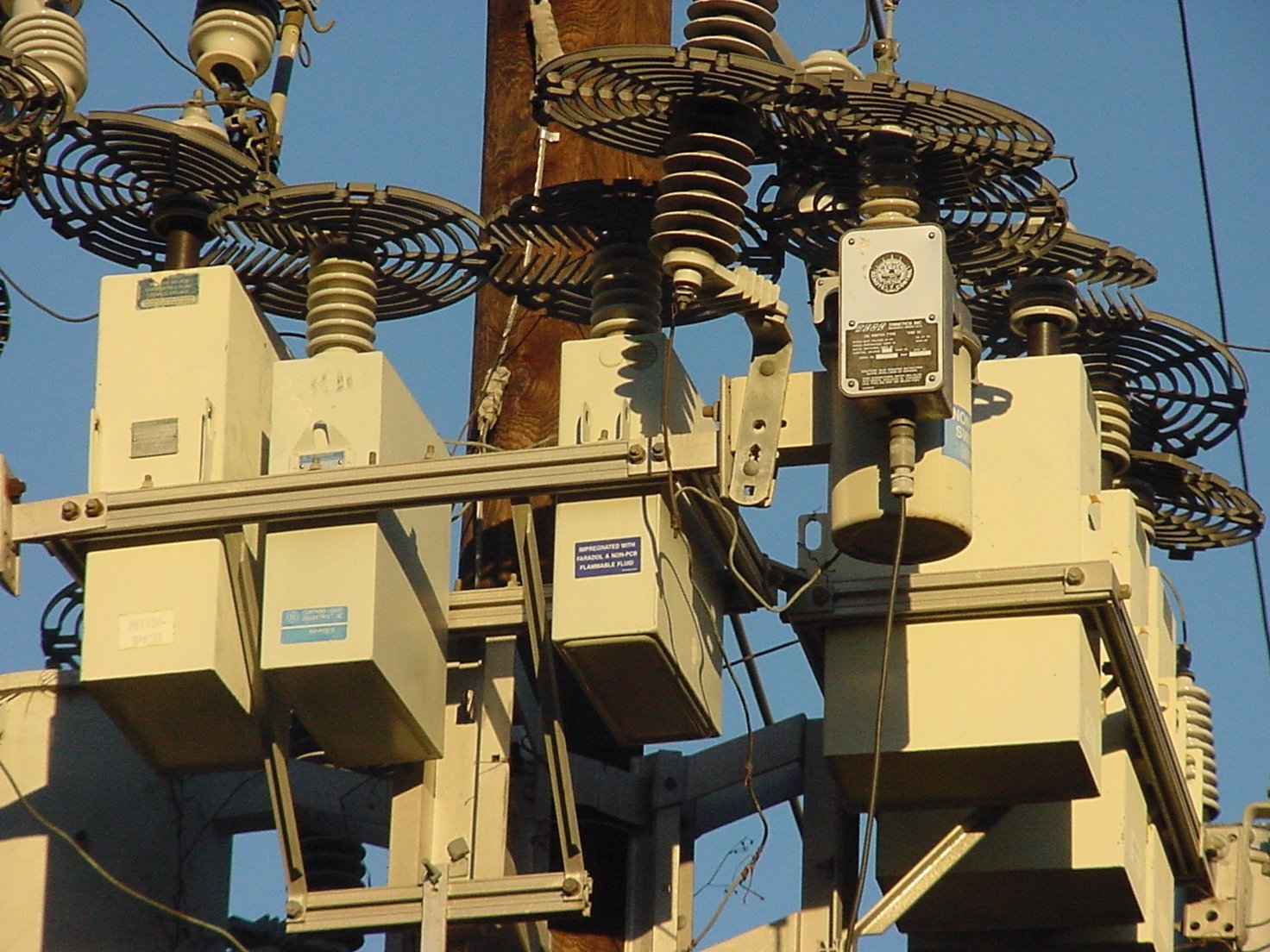## Power Factor and Harmonic Analysis

Course No.: PF101
CEUs 0.8Synopsis
This one day class takes you through the basics of power factor and harmonic analysis. How do you manage harmonics? Learn how to analyze harmonics, perform resonance calculations, understand IEEE 519, perform THD calculations and design harmonic filters. In addition, learn what to do about neutral harmonics which often require oversized neutrals and K rated transformers. Understand the interaction between harmonics and power factor correction capacitors

Description

POWER FACTOR CORRECTION
kW, kVA, kvar, PF Concepts. Leading and Lagging, Current Flow, Inductive Loads, PF and Load Magnitude, Vectors, Current and Voltage Angles

POWER FACTOR CALCULATIONS
Determining Var Requirements, Sizing the Capacitor, Switching Steps and Location at the Load vs. Closer to the Source, Capacitor Control

UTILITY RATE STRUCTURE AND ANALYSIS
Reviewing Utility Bills, Utility Rate Structure, Peak Demand, Demand and Power Factor Based Rates, Utility Rates in a Deregulated World

HARMONICS
Concept of Harmonics, Harmonic Spectrum, Sources of Harmonics, Non-Linear Loads, Harmonic Current Flow, Fourier Analysis, Graphical Representations of Harmonic Waveforms

HARMONIC RELATED PROBLEMS
Capacitor Failure, Fuse Interruptions, Equipment Over Heating, Metering Errors, Circuit Breaker Mis-Operation, Zero Crossing Issues

RESONANCE
Determining Parallel and Series Resonance, Effect of Source Impedance, Effect of Capacitor Size, Effects on Resonance, Impedance vs. Frequency Scans, Approximations of Parallel Resonance, Example Calculations of the Effect of Resonance Conditions

EVALUATING HARMONICS
Resonance Calculations, Total Harmonic Distortion (THD) Calculations, Effect of Parallel Resonance on THD, Effect of Source Strength on THD, Harmonic Sources and THD, Example Calculations of Source Impedance vs. THD

IEEE 519
Voltage and Current Distortion Limits, Point of Common Coupling, Enforcement of IEEE 519, Ratio of Load vs. Strength of the Utility System to Determine Limitations, Common Mis-Applications

THIRD HARMONICS
Switched Mode Power Supplies, 3rd Harmonics and Neutrals, Over sizing Neutrals, The use of Delta-Wye K-Factor Transformers, Over sizing Neutrals

CORRECTION OF HARMONIC RELATED PROBLEMS
Capacitor Operating Restrictions, Filtered Capacitor Banks, Over sizing Neutrals, Detuning Capacitor Banks

HARMONIC FILTER DESIGN
Tuning Frequency, Series Resonance, Sizing the Reactor, Evaluating the Capacitor Adequacy, Capacitor Voltage Stress, Filter Design Examples

CASE PROBLEM
Design of a 5th Harmonic Filter Tuned to the 4.7th